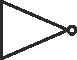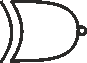# Basic Logic Gate Types

### - overview of the different types of logic gate and digital circuit building blocks that are available.

Digital electronics deals with the manipulation of numbers.

This is achieved by representing numbers in a digital form and using logic operations to provide the processing needed.

To achieve this there is a large variety of different types of logic gate and digital circuit building blocks that can be employed.

This digital circuitry forms the heart of many electronic products today. Even though many logic circuits or digital circuits are contained within large integrated circuits, the same basic functions apply. The only difference is the number of circuits contained within a large block.

## Basic logic gate and circuit types

There is a variety of standard building blocks or basic types of logic gate that can be used.

• Buffer:   Possibly not a proper type of logic gate in the strict sense, but sometimes a gate may be introduced to act as a buffer for a variety of reasons.Logic buffer gate symbol

Sometimes buffers may be introduced to give a higher output capability, to speed up the edge of a logic signal, or sometimes to add a small delay. In view of this, buffers are more widely used than might be expected at a first glance.
• NOT:   The NOT gate is possibly the simplest type of logic gate. It takes a single and inverts it. Using this logic gate or logic circuit, a logic "1" becomes a logic "0" and a logic "0" becomes a logic "1".Logic inverter circuit symbol

The NOT logic gate is an invaluable type of gate that is extensively used in logic circuits. Often two gates together may be used when no inversion is required but a small delay is needed. This technique can work when no buffers are present.
• AND / NAND:   The AND and NAND logic gates are normally viewed together because the output of one is the inverse of the other. The basic function of this type of logic gate is the same. In the AND type of gate, produces an output of "1" only when both inputs are "1", otherwise it produces a "0". The NAND type of logic gate produces the inverse of this.Logic AND gate circuit symbolLogic NAND gate circuit symbol

The AND and NAND gates are possibly the most widely used form of logic gate that are used. Of the two the NAND gate is the most widely seen.
• OR / NOR :   OR gates and NOR gates are another form of logic gate that form one of the basic building blocks of digital technology. The OR gate gives a logical "1" when one of the other input (or both inputs) is high.

Similarly the NOR gate is the inverse of this and goes low, "0" when one or the other input (or both inputs) is high.Logic OR gate circuit symbolLogic NOR gate circuit symbol

Like the NAND gate, the NOR gate is the more widely used of this pair of logic gates.
• Exclusive OR / NOR:   An exclusive OR or NOR type of logic gate is used where an output change is only required where one or the other input is high and not both.Logic Exclusive OR gate circuit symbolLogic Exclusive NOR gate circuit symbol

Although the more familiar standard type of OR or NOR gate is more widely used, there are some circumstances where the exclusive OR or NOR is required.
• RS Flip-Flop:   The initials of the RS flip flop stand for Set-Reset. This type of logic gate or circuit has two inputs, the set and reset. As might be expected, this type of logic circuit is set when one input is triggered and reset when the other is triggered. Read more about the RS Flip Flop
• D-Type Flip Flop:   The D-Type flip flop could be termed as a form or type of logic circuit. It is a form of flip flop that transfers the input data to the output on the edge of the clock signal. It is a particularly useful form of flip flop that finds many uses in a variety of applications.
• J-K Flip Flop:   The J-K flip-flop is the most versatile of the basic flip-flops and it can be configured to operate in a variety of modes.
• Counter:   Counters are widely used in various forms of logic circuit. Essentially they take in a train of pulses and dependent upon their division ratio, the output a pulse after a set number of pulses have entered.

More Digital Logic and Embedded Topics:
FPGA programming     Embedded systems     How a computer works     Logic circuit design basics     Logic / circuit design guidelines